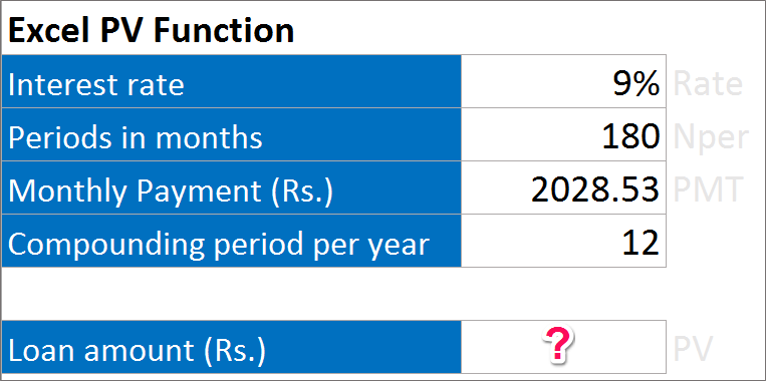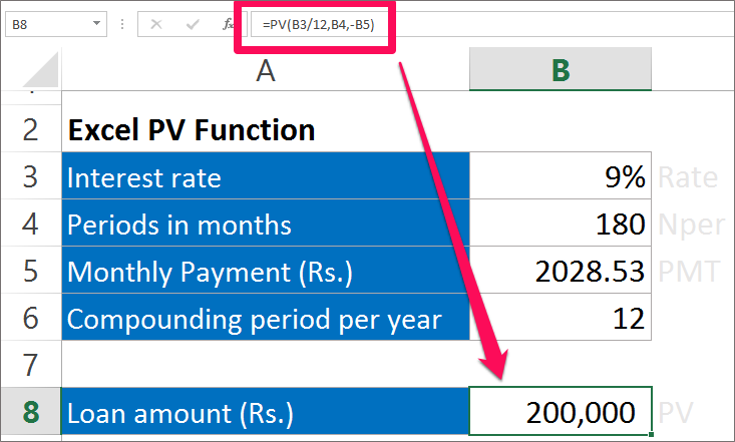Need Help? Chat with us

# PV Function | Present Value formula in Excel in Hindi

## PV Function | Present Value formula in Excel

PV function denotes the Present Value of the investment. It will help us to calculate the present value of the future investments. The PV function calculates the present value for today when you know the annuity value yearly or per month, rate and number of years. PV function will tell you how much you need to save today in order to spend a particular amount in the future.

Note: Present Value will always be less than the future value.

## Special Offer for First Time Users

If you are the first time user and want to learn Microsoft office skills, then you can avail this special offer discount on your email.The Formula for calculating Present Value (PV function)

PV(rate, nper, pmt, [fv], [type])

Where,

PV stand for the Present value of investment.

Rate stand for the rate at which you will invest the money.

Nper stand for the time period for which you are going to invest your money.

FV stand for the Future value of the investment.

Type stand for the time at which the investment is made at the beginning of the period or at the end of the period.

The argument in the Box bracket are optional

### Example of PV Function

If we make a monthly payment of Rs 2028.53 for 15 years loan with annual interest rate at 9%. How much will be the present value of the loan amount?Solution:

Here, Rate: 9%

Nper: 15*12 = 180

PMT: Rs 2028.53

The other arguments are optional.